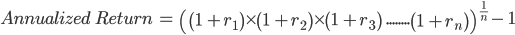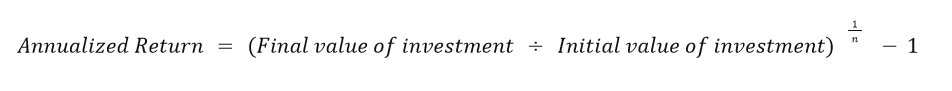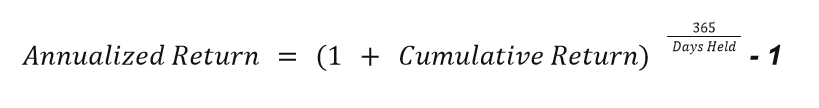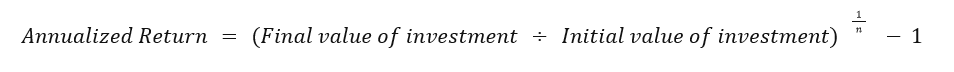# Annualized Total Return

It is a geometric average of annual company returns computed to indicate how much investors would receive if the annual investment return was compounded.

The annualized total Return is the amount of money invested over time. It is a geometric average of annual company returns computed to indicate how much investors would receive if the annual investment return was compounded.Calculating a company's annualized Return is critical for investors since it reveals an investment's average Return (or loss) over 12 months and is typically represented as a percentage.

Investors often aim to make wise investment decisions, such as where to invest for the best Return. Calculating an investment's annualized total Return is one method that would assist investors in making selections that align with their expectations.

Using this metric, Investors can compare the annualized total Returns of various investments such as stocks, bonds, real estate, and other assets. Investors can even compare the annual returns of different investments over different periods.

## importance

The annualized total Return is used because it helps investors determine how much they earn for each investment yearly and compare how well each investment performs.The annualized Return demonstrates that the amount of an investment's losses/ gains in one year depends on the number of losses/gains from previous years since it considers the compounding method.

By determining the total Return, investors can find the following:

• Investors can determine the investment with higher total returns in the market, so they better understand what kind of business generates the highest annual Return
• Investors can ensure that they put their money into a suitable investment that generates an excellent annual return and meets their expectations.
• Investors can evaluate the success of their investments. For example, if investors have extra funds and are willing to reinvest their money, they could reinvest in one of their previous investments with a higher success rate.
• Investors can evaluate the success of their investments compared to other businesses. They may notice other investment opportunities with higher returns and success rates.
• Investors can understand the business annual returns fluctuations with the risks associated with returns volatility.Suppose an investor holds stocks in TYL and LYC companies for four years. Then, the annual Return of companies' stocks is as follows:

1. Stocks in TYL company: 5%, 9%, 7%, 14%.

2. Stocks in LYC company: 6%, 8%, 7%, 8%.

By comparing the return rate of the companies, it is evident that the fluctuation under TYL Co. is more significant than LYC Co., which investors should consider in their investment decisions.

Remember that a change in investment price doesn't reflect the total Return of a single year.

Investors use annual business returns for many years rather than annual returns for a single year since it isn't the best approach to figure out possible price variations, adverse changes, or investment volatility.

## Formula

Annual Return is the average of an investment's earnings over time. Therefore, calculating the yearly Return needs the years and the investment's Return.1. First method:

If an investor is given the yearly rate of Return for each year of the investment period, the annualized total Return can be determined using the formula below.Where,

N: Number of years that are being measured. For example, when you try to apply this ratio for calculating the Return of business over six years, the "N" value would be "6".

R: Yearly rate of Return

2. Second method

If an investor has the initial and final value of an investment in dollars, An annualized return can be calculated by the following formula:• The final value is the value of a business at the end of a period that is calculated.
• The beginning value is the value of the company at the beginning when an investor invests.

3. Third method

Suppose an investor has a cumulative return for a particular time. In that case, even if the period is a defined number of days, An annualized return can be calculated using the following formula.According to Global Investment Performance Standards (GIPS), The business cannot annualize its performance if it runs for less than one year.

In other words, the performance of an investment that has been in operation for six months cannot be reported to be annualized.

It is not permissible to declare that the investment has an annualized return of 20% because the business runs for six months with 10% annualized returns. Forecasting future returns must be based on actual data (history records).## Examples

Annualized total returns give investors an image of how much earnings they get from their business over several years. So, most investors and companies use this measure to ensure that their business is going in the right direction.

Investors utilize this indicator to determine how much they will earn in the following years based on their earnings in the previous years. Therefore, investors can't predict future earnings based on a single year's financial statement.

However, knowing how to calculate annual returns is a must to get accurate financial information about your business. The following example includes the way of calculating this measure:

1. Suppose an investor holds stocks for four years. The annual Return of a mutual fund is as follows:

First year: 4%.

Second year: 8%.

Third year: 6%.

Fourth year: 13%.

As shown in the example, the yearly return rate is given for each year, so the following formula is being used:Annualized return rate = [((1 + .04) * (1+.08) * (1+.06) * (1+.13)) ^ ¼] -1

= ((1.04 * 1.08 * 1.06 *1.13)^¼ ) -1

= 1.077 -1 = 0.077 or 7.699%

Hence, the investor earns a return of 7.69 each year for the four years the stocks were held.1. Suppose an investor bought 150 shares in 2018 for \$14 each and planned to keep them for 4 years, until 2021. Each year, the investor gets \$2.50 in cash for each share. The investor decides to sell all shares at \$17 after four years. What was the rate of Return in the four years that the investor-owned the claims?

Let's first highlight that the investor is given the initial and final value of the investment in dollars so that the following formula would be used.First, calculate the initial and final value of the investment and then apply it to the formula.

Initial value of investment = \$14 * 150 = \$2,100

Cash received as dividends over two-year period = \$2.50 * 150 * 4 = \$1,500

Value from selling the shares = \$17 * 150 = \$2,550

Hence, the final value of investment = \$1,500 + \$2,550 = \$4,050Now, apply the value in the formula:= (( \$4,050 / I \$2,100) ^ (1 / 4) -1) * 100

= (1.93^0.25) * 100

= (1.178 -1) * 100 = 0.178 * 100 = 17.84%

Hence, the investor earns 17.84% each year for the four years the stocks were held.2. Assume an investor holds stock for 580 days and receives a cumulative return of 26.94%. What is the annualized rate of Return?

As shown in the question, the investor has a cumulative return for 580 days, so the following formula is used:= [(1 + 26.94%) ^ (365 / 580) - 1] * 100

= ((1.269 ^0.629) - 1) * 100

= (1.162 - 1) * 100

= 0.162 *100 = 16.197%

Hence, the investor earns 16.197% for 580 days the stocks were held.

## Benefits and limitations

Even though annualized ROI provides an overview of business performance over time, it has some limitations in some cases. Therefore, knowing its limits and benefits is necessary as it points out when its accuracy decreases and increases.Investors can rely on these measures before investing in a particular company. For example, using this metric, investors could evaluate the success of a specific investment compared to its competitors and determine the investment with higher total returns.

Consequently, Investors can ensure that their money is placed in a high-profit investment that yields an annual return and thus satisfies their expectations.

Additionally, investors can assess the success of their old investments. If investors are ready to reinvest, they could do so in one of their old investments with a better success rate.However, annualized ROI can be misleading in some cases of new investments that operate for less than a year or in times of worsening economic conditions. Generally, new mutual funds operate poorly initially, even in a good economic environment.

Investors cannot rely on short history data to annualize company returns and judge a company's performance. A fund's returns may be low for a brief period due to poor market circumstances and economic conditions.

For example, annualized the Return of investments that launched a few months before COVID-19 would be challenging due to the market conditions, shut-down, work from home mandates that caused a substantial impact on customer demands.Applying annualized returns of start-up companies for investment purposes will prove incorrect and deceptive, which means relying on short history data may generate inaccurate returns and lead to wrong investment decisions.

In other words, the accuracy of annualized ROI could show some limitations; company history and data are insufficient for analysis. Therefore, the accuracy of annualized ROI depends on the available data; the more, the better.

## Annualized Total Return Vs. Average Return

Annualized and average returns may look similar, but they are not. Understanding these differences and the pros and cons of these two calculations can help you decide which to use to analyze your investment.Both measures are often used to judge how well a business is doing. However, average returns ignore compounding returns, a significant consideration in annualized returns calculation.

Simple averages can only be found when the numbers are not related to each other. The annualized Return is used because of compounding; the amount of money lost or gained on an investment in one year depends on what happened in the other years.

The average Return is the annual Return on an investment over a year and is expressed as a percentage of the initial investment. It is regarded as a gain or loss on the initial investment, depending on whether the Return is positive or negative.

The rate of return changes depending on the level of risk involved in the investment. The average annual Return is calculated by dividing the total Return over time by the number of periods.

In other words, the average Return is about adding all the returns together but does not consider compounding or allow for comparing mutual funds or stocks.An annualized return, known as the "geometric average," is the annual rate of Return on an investment that assesses how much is lost or gained over a while when compounding is considered.

It can also give you a better idea of how different stocks have been traded over time and help you make investment decisions.

For example, a fund makes the following returns:

First year: 15%

Second year: 23% (loss)

Third year: 18%

Fourth year: 14%

Compute the average Return for the fund

Average return = (15% + (-23%) +18% + 14%) / 4 years

= 24% / 4 years = 6%

However, the annualized Return for the same investment would be different, as shown below:

Annualized Total Return = ((1+15%) * (1 + (-23%)) * (1 + 18%) * (1 + 14%))^¼ -1

= ((1.15) * (.77) * (1.18) * (1.14))^1/4 – 1 = 1.045 -1

= 0.045 * 100 = 4.47%

## Key takeaways

1. It is a geometric average of annual business returns that indicates how much investors would receive if annual investment returns were compounded.
2. Using the information produced from the annualized return formula, an investor can evaluate the performance of an investment by comparing its Return to those of similar assets.
3. The annualized total Return of various investments such as stocks, bonds, and real estate can be compared. Investors can also compare the annual returns of different investments with varying durations.
4. It is important to note that the annual Return of a single year doesn't show the investment price fluctuation.
5. Investors use annual business returns for many years rather than annual returns for a single year since it isn't the best approach to figure out possible price variations, adverse changes, or investment volatility.
6. Two factors are required to calculate the annualized rate of Return: the returns for a specific period and the number of years the investment was held.
7. Annualized and average returns may look similar, but they are not. Both measures are often used to judge how well a business is doing.
However, average returns ignore compounding returns, a significant consideration in annualized returns calculation.### Everything You Need To Master Valuation Modeling

To Help You Thrive in the Most Prestigious Jobs on Wall Street.